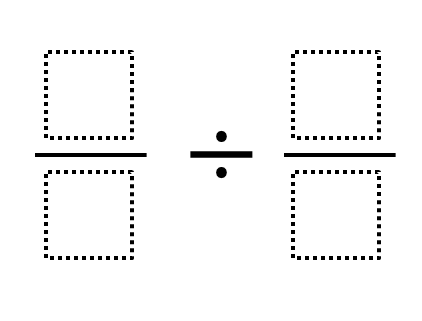Home > Grade 6 > Undefined Quotient with Fraction Division

# Undefined Quotient with Fraction Division

Directions: Give at least two different examples where the quotient is undefined by filling in the boxes with whole numbers 0 through 9, using each number at most once for each example.### Hint

What does undefined mean?
What operation could produce undefined values?

Source: Daniel Luevanos

## Converting a Fraction to a Decimal

Directions: Using the digits 1 to 9, at most one time each, place a digit …

1.I think the third solution is wrong. When you invert the divisor #/0, it becomes 0/# so you have #/# x 0/# which is zero, not “undefined”.

•I understand your reasoning, Patsy, but I don’t know if I would agree with you that the third solution is wrong.

Let’s imagine that we look at the example “4/5 divided by 3/0”. There are a two ways that I might interpret this question:

1.) We could think of the fractions as decimals. In doing so, we could interpret this example as “0.8 divided by undefined” which would lead to a result of undefined.

2.) We could think of the example as “4 divided by 5 divided by 3 divided by 0” which would have a result of undefined.

3.) Just to check my work, I typed the question in a calculator and the message “Cannot divide by zero” popped up.

2.Just a typo…”quotient” is spelled incorrectly in the directions.

Thanks for being a great resource for interesting math problems!

•Fixed. Keep ’em coming. We all benefit from tightening them up.